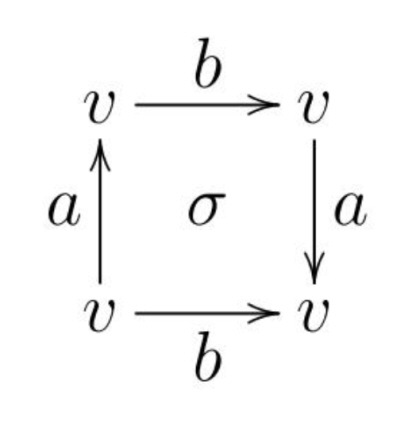# What does it mean to attach a cell to a space by a map?

I am starting to study for my algebraic toplogy exam and a lot of the problems sound like:

let $Y_p$ be the space obtained by attaching an $(n+1)$-cell to $S_n$ by a map of degree $p$, where $p$ is a prime.

The thing is I do not really understand what attaching a map to a space means. Can someone please explain or give a specific reference?

• This is explained in pretty much any textbook on the subject. You should browse a couple, at least, really! – Mariano Suárez-Álvarez Jan 31 '15 at 9:05

This question is in my view best answered by explaining the general situation of attaching a space $X$ to a space $B$ by means of a map $f: A \to B$ defined on a subspace $A$ of $X$ to form a space $Y= B \cup _f X$, called an adjunction space. A detailed account is in Topology and Groupoids.

The advantages of adjunction spaces over more general identifications are that they are easily described as sets, the disjoint union of $B$ and $X \backslash A$, but we think of it as points of $A$ identified into $B$ by means of $f$. The topology is the identification topology with respect to the function $X \sqcup B \to B \cup_f X$. An important property is that we obtain a pushout diagram:

$$\matrix{A &\to &B \cr \downarrow & & \downarrow \cr X & \to &B\cup _f X}$$ so that maps $B \cup_f X \to Z$ are determined by maps $X \to Z , B \to Z$ which "agree on $A$", i.e. when composed with the maps $A \to X, A \to B$. This illustrates the point that one of the reasons for constructing this topology on $B \cup_f X$ is to be able to define continuous functions on it.

Other useful properties of the adjunction space are that if $A$ is closed in $X$, then $B$ is closed in $Y=B \cup_f X$, and also $X \setminus A$ maps homeomorphically into $Y$.

Such properties come into their own when defining a CW-complex by starting with a discrete space and then attaching cells in order of increasing dimension. This allows the construction of continuous maps by order of increasing dimension.

One origin of the notion of attaching a cell is the desire to get away from the awkwardness of simplicial subdivision. For example the standard diagram for the Klein Bottle is the following:Describing this in terms of simplices is complicated and disguises the geometry. But we can easily describe it as attaching a disc $D^2$ to a wedge $S^1\vee S^1$ of two circle by means of a map whose class in the fundamental group of $S^1\vee S^1$ is $-b+a +b +a$. Note that we would like to write this element as $\partial \sigma$ but that is not possible in the usual abelian framework of chains. For more discussion of that, see this presentation.

The earliest paper on adjunction spaces that I have studied is by J.H.C. Whitehead, "Note on a theorem due to Borsuk", Bull. Amer. Math. Soc. vol. 54 (1948) pp. 1125-1132, and the notion was used extensively in his paper "Combinatorial homotopy I" which defined the notion of CW-complex.

So I believe part of the "meaning" of a term such as "attaching a cell" is to understand how it arose and that the concept was dragged "out of the dark" after quite a long process.

• Can you please update the link you have given? It appears broken. Thanks! – Iulia Feb 7 '15 at 21:46
• Thanks: correction made. You can also look at my book "Topology and groupoids" advertised at pages.bangor.ac.uk/~mas010/topgpds.html . – Ronnie Brown Feb 11 '15 at 11:13

This means that we are taking a particular quotient space. For your example, let $f:\partial D^{n+1}\to S_n$ be a map of degree $p$, where $D^{n+1}$ is the unit $n+1$ ball. The space being constructed is the quotient of the disjoint union of $S_n$ and $D^{n+1}$ by the equivalence relation $x\sim f(x)$ for $x\in \partial D^{n+1}$.

If you have a wire ring sitting on the table ($S^1$), and a circular disc in your hand (a $2$-cell), you could attach the disc to the ring by gluing the disc's edge to the wire. But you would have to start by taking a point on the edge of the disc and deciding where on the wire ring you would glue it to. So in this way, you are creating a map from points on the edge of the disc to points on the wire ring. If you now let your circular disc be some kind of elastic material, you can get more interesting maps then if the disc is rigid paper.

Your situation is a higher dimensional analogy to this.

Also, in your question you say "what attaching a map to a space means". That does indeed sound a little nonsensical, and it's not what the problem is saying. It talks about attaching one thing to another thing using a map, not attaching a map to anything.

• Yes, thank you for the observation; when I formulated the question in the text I stated it incorrectly. The title is correct though. – Iulia Jan 31 '15 at 23:56